# TikZ: complicated paths, e.g. for complex integrals

I would like to illustrate lecture notes on complex analysis, which by its nature is a lot about how the lines of integration are running through the complex plane. However, I'm having trouble finding a proper solution with tikz to do so.

Take for example the following diagram, which should become a path coming from the upper right middle, then joining the circle, exiting at the lower right middle again:

\tikz{
\draw[help lines] (-2,-2) grid (2,2);
\draw (0, 0.1) -- (2, 0.1);
\draw (0,-0.1) -- (2,-0.1);
\draw (0,0) circle (1);
}I would now like to cut out the shapes that do not belong to the individual paths, so that it becomes something like• I've been looking for something like \clip[inverse], but that apparently that doesn't exist.
• I also tried calculating the intersections by hand (i.e. Mathematica) to and then using arcs and other nasty things to do the trick, but after a few diagrams I thought this can't be it.
• The tikz manual didn't help (which is surprising to be honest). Am I overlooking some feature that does what I want?

Problem solved, basically using an inverse clipping technique. See my response below.

A) With Jake's code (I hope to use correctly this code)

Update version 1 (I added a cip on the all path to color the picture )

\documentclass{article}
\usepackage{tikz}
\usetikzlibrary{calc}

\begin{document}

\begin{tikzpicture}[remember picture,overlay]

\tikzstyle{reverseclip}=[insert path={(current page.north east) --
(current page.south east) --
(current page.south west) --
(current page.north west) --
(current page.north east)}
]
\begin{scope}
\path[clip] (1,-0.1)--(2,-0.1)--(2, 0.1)--(1,0.1)%
arc({asin(0.1)}:{360-asin(0.1)}:1cm)--cycle;
\fill[blue!20] (-1,-1) rectangle (2,1);
\end{scope}

\path[clip] (0,0.1) --(0,-0.1)--(2,-0.1)--(2, 0.1)--cycle[reverseclip];
\draw[clip] (0,0) circle (1) [reverseclip];
\draw[black] (0, 0.1) -- (2, 0.1);
\draw (0,-0.1) -- (2,-0.1);
\end{tikzpicture}
\end{document}B) Update version 2 With tkz-euclide

 \documentclass{scrartcl}
\usepackage{tkz-euclide}
\usetkzobj{all}

\begin{document}

\begin{tikzpicture}
\tkzDefPoint(0, 0.1){A}
\tkzDefPoint(0, -0.1){B}
\tkzDefPoint(2,- 0.1){C}
\tkzDefPoint(2, 0.1){D}
\tkzDefPoint(0,0){O}
\tkzInterLC[R](A,D)(O,1 cm)  \tkzGetPoints{I1}{I2}
\tkzInterLC[R](B,C)(O,1 cm)  \tkzGetPoints{J1}{J2}
\tkzDrawArc(O,I2)(J1)
\tkzDrawSegments (I2,D J1,C)
\tikzset{compass style/.append style={<->}}
\tkzDrawArc[R with nodes,color=blue](O,1.2cm)(I2,J1)
\end{tikzpicture}

\end{document}• The problem with Jake's code is that it doesn't clip the path, but also how it is displayed, in the sense that "it cuts one-dimensional lines in half". I'll have a look at Euclid and see whether it's possible to decorate the path there (since I need arrows along the path). Thank you. – David Dec 5 '11 at 17:48
• Perhaps Jake can give a better answer than mine. Now tkz-euclide' helps you to get all the points after you can use normal tikz code to decorate the path. I don't know what you want to draw but I modifiy my answer with an arc and arrow to show you some possibilities. – Alain Matthes Dec 5 '11 at 18:28
• I've edited my question, adding the thing that I actually need. – David Dec 5 '11 at 19:25

This is actually pretty easy using some math... If you want the lines at the right hand side to be 0.2 appart (as in your example) one 0.1 above and one 0.1 below a straight line to the right. Then the angles where they intersect the circle are asin(0.1) and 360-asin(0.1) respectively. So the following code generates the picture:

\documentclass{article}
\usepackage{tikz}
\begin{document}
\begin{tikzpicture}
\draw (1,0) -- ++(-1,0) arc({asin(0.1)}:{360-asin(0.1)}:1cm) -- ++(1,0);
\end{tikzpicture}
\end{document}


Looking like this:Edit (after Altermundus' comment): For the picture as is it doesn't really matter where the center is. If you add additional stuff to the same picture environment or use overlay it can become important. The distance between the horizontal lines is dependent on the radius of the circle, not on whether or not it depends on other objects. It looked to me like scaling the distance between the lines was something desirable. If this is not the case it can be easily taken care of by dividing by the radius of the circle. As far as the placement of the center goes, if this is important it can also easily be dealt with. If we parameterize the entire thing a little more we get the following:

\documentclass{article}
\usepackage{tikz}
\begin{document}
\def\myCircleWithLines#1#2#3#4{
%#2: Length of the line segments on the right
%#3: y offset of line 1
%#4: y offset of line 2
\begin{tikzpicture}
\path[draw] (#1+#2,#3) -- ++(-#2,0) arc({asin(#3/#1)}:{360-asin(-#4/#1)}:#1 cm) -- ++(#2,0);
\path[fill] (0,0) circle (1pt);
\end{tikzpicture}
}
\myCircleWithLines{1}{1}{.1}{-.1}\\
\myCircleWithLines{2}{1}{.1}{-.1}
\end{document}


Which results in:Note that the \path[fill] (0,0) circle (1pt); is only present to demonstrate the fact that the center is actually located at (0,0).

• You don't get the same picture. The center is not exactly at (0,0) and you don't have the same horizontal lines. if the picture depends of other objects, you need to add some calculations but perhaps I'm wrong. – Alain Matthes Dec 5 '11 at 9:25
• @Altermundus , yes but you can just as easily put in coordinate or convert it to my code where specific points are used, his main idea of showing how to get the correct placement of the clipped circle is the key point here. – zeroth Dec 5 '11 at 9:42
• The problem with calculating everything yourself is it doesn't go well with more general pictures. For example, the "hose" to the right might be bent in order to go around a singularity, or the circle might be a 45° rotated ellipse. – David Dec 5 '11 at 17:59
• @David: everything can be done with math. Although in a lot of cases it might not be a very good idea. – Roelof Spijker Dec 5 '11 at 21:07
• @wh1t3, not using math (what am I saying, trigonometry) is kind of why I want to use TikZ. – David Dec 5 '11 at 23:34

I found an answer to pretty much exactly what I wanted to do, with very little workaround character. It pretty much is an inverse clip command. The answer is motivated by the yellow part of the example found at http://www.texample.net/tikz/examples/venn-diagram/

The idea is dividing up the single parts of the diagram in their own scopes, i.e. one diagram for the lines, one for the circle. Each of these then gets appropriate clipping. A small example:

\begin{tikzpicture}
% Draw the full lines dashed to visualize what is clipped later
\foreach \x in {-0.5,0.5} {
\draw[dashed] (0,\x) -- (2,\x);
}

% Draw the two lines.
% Even odd rule is explained in the manual. Here, it's helping us to punch holes into a shape. For more complicated geometries, there's the nonzero rule.
\begin{scope}[even odd rule]

% This is the clipped area drawn in gray to visualize where drawing will be possible later. It's the same shape as the \clip below.
\draw[fill=black!10,draw=none] (-2,-2) rectangle (3,2) (0,0) circle (1);
% Draw a large rectangle, the subtract the parts of the second shape given (circle) from it. The result is a rectangle with a hole in it.
\clip (-2,-2) rectangle (3,2) (0,0) circle (1);
% Done. We can now draw in the gray area.
\foreach \x in {-0.5,0.5} {
\draw (0,\x) -- (2,\x);
}
\end{scope}
\end{tikzpicture}


Result:The same technique again for the circle, this time we're cropping out a rectangle:

\begin{tikzpicture}
\begin{scope}[even odd rule]
\draw[fill=black!10,draw=none] (-2,-2) rectangle (3,2) (0,0.5) rectangle (3,-0.5);
\clip (-2,-2) rectangle (3,2) (0,0.5) rectangle (3,-0.5);
\draw (0,0) circle (1);
\end{scope}
\end{tikzpicture}


Result:And finally, the thing that I wanted to achieve in my initial post, only that this time I don't need Gimp to edit out the unwanted pieces :-)

\begin{tikzpicture}
\draw[black!20] (-2,-2) grid (3,2);

% draw the two lines
\begin{scope}[even odd rule]
\clip (-2,-2) rectangle (3,2) (0,0) circle (1);
\foreach \x in {-0.5,0.5} {
\draw (0,\x) -- (2,\x);
}
\end{scope}

% draw the circle
\begin{scope}[even odd rule]
\clip (-2,-2) rectangle (3,2) (0,0.5) rectangle (3,-0.5);
\draw (0,0) circle (1);
\end{scope}
\end{tikzpicture}An example of this applied in a complex analysis problem:• That's excellent! Would you mind adding the code for your final (very impressive) example, or at least a snippet showing how you get the arrows on the paths? – Jake Dec 7 '11 at 4:12
• It's all just decorated paths and the technique from above applied. The code is rather lengthy and would pretty much blow up my post. I've uploaded the snippet, you can see it there. (I use a bunch of includes grown over the years, but there shouldn't be too many custom commands in there.) stupidname.org/files/temp/temp_2011_12/path – David Dec 7 '11 at 5:40

If you really do not care where you will draw these things (i.e. do not want to know the exact coordinates, this can be done in one line: \draw (2,0) -- ++(-2,0) arc (10:350:3cm) -- ++(2,0);. However I do not think that this will be the case for you.

I have here shown you how it can be done (without clipping), in the case of circular diagrams there really is no need for clipping, tikz does a pretty good job, besides you skip a lot of scope environments.

So the code, I have attached also the minimal example which is the same as the other. Please notice the use of easy customizable angles and radius' of the diagram. Change \myRadius and everything is changed. This will help you in the long run!

\documentclass{article}
\usepackage{tikz}
\usetikzlibrary{calc}
\begin{document}
\begin{tikzpicture}
\draw (2,0) -- ++(-2,0) node[above right] {$C_1$} arc (10:350:3cm)  node[below right] {$C_2$} -- ++(2,0);
\end{tikzpicture}

\def\myAngle{10}
\begin{tikzpicture}
\draw ($(C1)+(2,0)$) --
(C1) arc (\myAngle:360-\myAngle:\myRadius) coordinate (C2) -- ++(2,0);
\node[above right] at (C1) {$C_1$};
\node[below right] at (C2) {$C_2$};
\end{tikzpicture}

\end{document}


I wrote a library named avoidpath that is available on Github. With this small library we can do :

\documentclass[border=7mm]{standalone}
\usepackage{tikz}
\usetikzlibrary{avoidpath}

\begin{document}
\begin{tikzpicture}
\draw[help lines] (-2,-2) grid (2,2);
\end{tikzpicture}
\end{document}This library adds one to path style called avoid that has two modes :

• when the pole is on the segment :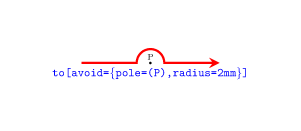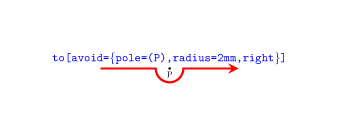• and when the pole is out the segment :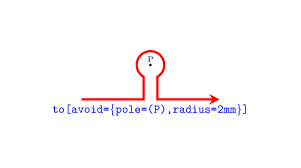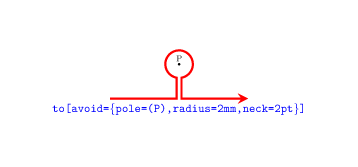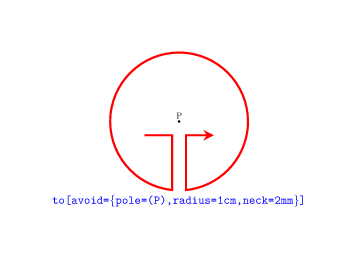So for example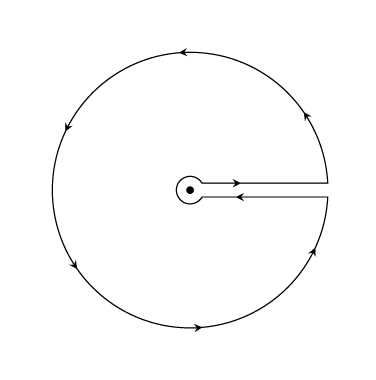is obtained by

\draw [avoid={pole={(P)},neck=2mm},with arrows=21mm]
(0,0) node[scale=3](P){.}
`